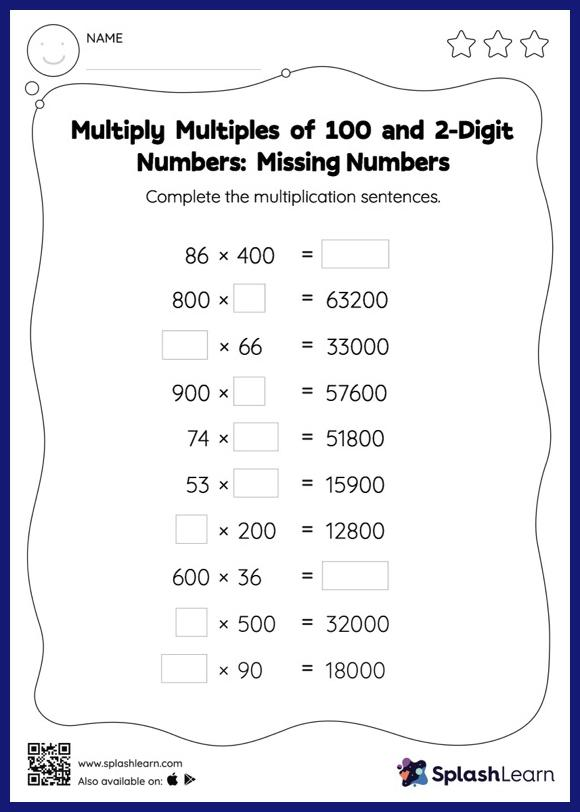# Multiply Multiples of 100 and 2-Digit Numbers: Missing Numbers Worksheet

Home > Multiply Multiples of 100 and 2-Digit Numbers: Missing NumbersStudents love it when they get to multiply numbers by 10, 100, or their multiples. They use the pattern of zeros to find the product. In multiply multiples of 100 and 2-digit numbers worksheet, they use the same concept to find the missing number in multiplication sentences. This worksheet is about practicing with the horizontal format in which numbers are written side by side. To develop flexibility with numbers and operations, students need to have enough practice in this format and not just rely on the vertical/column method.